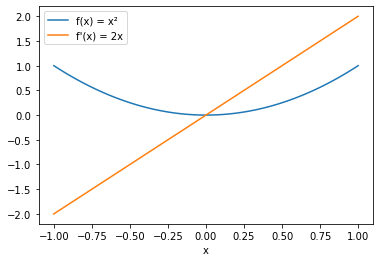# Simple Derivative¶

Suppose we want to differentiate the function $$f(x) = x^2$$. For this function we can easily state its analytical derivative $$f'(x) = 2x$$.

Instead of implementing the analytical derivative by hand, we want to have it computed algorithmically. Let’s say we are interested in the function value $$f(x)$$ and its derivative at $$x=0.5$$.

First we need to implement a function f which computes $$f(x)$$. We are already able to compute $$f(0.5)$$.

:

def f(x):
return x**2

print ("f(0.5) = {}".format(f(0.5)));

f(0.5) = 0.25


To generate a function which computes the derivative, we use the derivative function from the pyADiff package. This returns a function for the derivative which we here call df. Now we are able to compute $$f'(0.5)$$

:

import pyADiff

print("f'(0.5) = {}".format(df(0.5)));

f'(0.5) = 1.0


This result can be verified using the analytical derivative $$f'(0.5) = 2 \times 0.5 = 1$$.

It might be more convenient to see the functions $$f$$ and $$f'$$ as graphs. Therefore the packages numpy and matplotlib.pyplot are included.

:

import numpy as np
import matplotlib.pyplot as plt


Now we initialize x as 100 points between -1 and 1. And calculate the function value $$f$$ and its derivative $$f'$$ at each of the points in x. Note the wayf and df are called repeatedly. While for f the numpy vectorization would work as expected and would return all of the inputs x squared, pyADiff would interpret f as a function $$f : \mathbb{R}^{100} \to \mathbb{R}^{100}$$, where $$f_i(x) = x_i^2$$ and would return the jacobian of $$f$$.

Therefore we explicitly call f and df for each x_i in a loop here.

:

x = np.linspace(-1, 1, 100)

y = np.array([f(x_i) for x_i in x])
dy = np.array([df(x_i) for x_i in x])


The last step is to plot the function $$f$$ and its derivative $$f'$$ over x.

:

plt.plot(x, y, label="f(x) = x²")
plt.plot(x, dy, label="f'(x) = 2x")
plt.xlabel('x')
plt.legend();